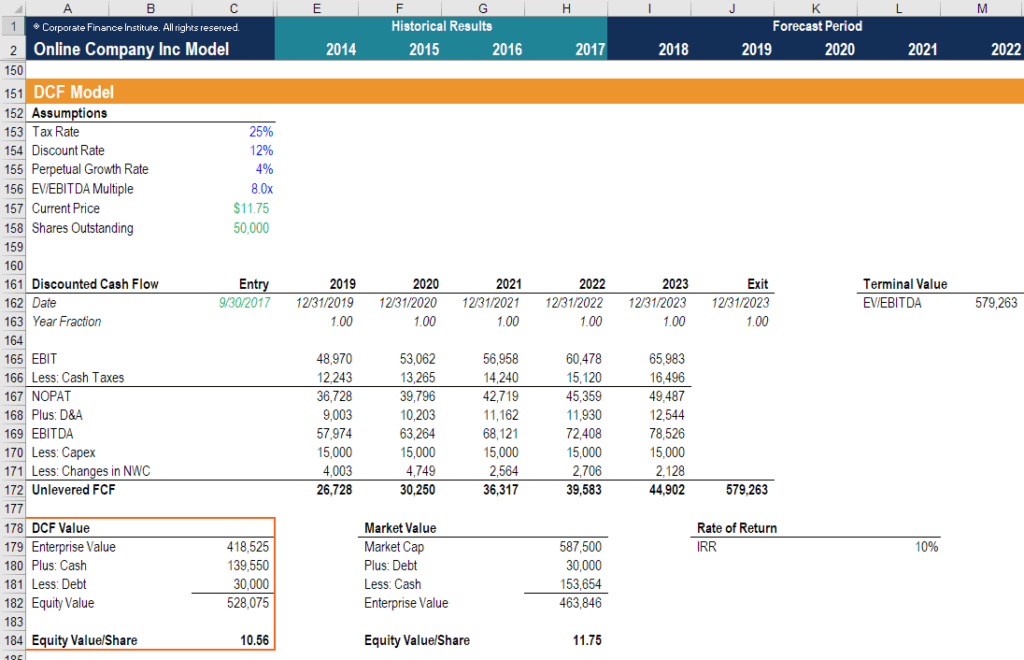# Terminal Growth Rate

How the terminal growth rate is used in valuation

## What is the terminal growth rate?

The terminal growth rate is a constant rate at which a firm’s expected free cash flows are assumed to grow at, indefinitely. This growth rate is used beyond the forecast period in a discounted cash flow model, from the end of forecasting period until perpetuity, we will assume that the firm’s free cash flow will continue to grow at the terminal growth rate, rather than projecting the free cash flow for every period in the future.### A view of multi-stage growth rates

When making projections for a firm’s free cash flow, it is common practice to assume there will be different growth rates depending on which stage of the business life cycle the firm currently operates in.

Typically, we construct a three-staged growth model to project a firm’s free cash flows and determine said firm’s value at each level of maturity:

#### #1 Expansion stage growth rate

We assume a high growth rate (usually over 10%) for business in its early stage of expansion. The business has established its position in the industry and is seeking to increase its market share. As such, it will experience a rapid growth in revenue and, thus, free cash flow.

#### #2 Decelerated growth stage

The rapid-growth stage is often followed by a relatively decelerated growth stage, as the company will likely struggle to maintain its high growth rate due to the rising competition within the industry. The business will continue to grow, but no longer at the substantial growth rate it had previously experienced.

However, as the company evolves closer to maturity, it is expected to hold a steady market share and revenue. We often assume a relatively lower growth rate for this stage, usually 5% to 8%.

#### #3 Mature stage growth rate

We assume the company will grow at the terminal growth rate when it reaches a matured stage. At this stage, the company’s growth is minimal as more of the company’s resources are diverted to defending its existing market share from emerging competitors within the industry.

A positive terminal growth rate implies that the company will grow into perpetuity, whereas a negative terminal growth rate implies the discontinuance of the company’s operations. The terminal growth rates typically range between the historical inflation rate (2%-3%) and the average GDP growth rate (4%-5%) at this stage.

A terminal growth rate higher than the average GDP growth rate indicates that the company expects its growth to outperform that of the economy forever.

### Application of the terminal growth rate

The terminal growth rate is widely used in calculating the terminal value of a firm.

The “terminal value” of a firm is the net present value of its cash flows at points of time beyond the forecast period. The calculation of a firm’s terminal value is an essential step in a multi-staged discounted cash flow analysis and allows for the valuation of said firm.

In a Discounted Cash Flow DCF Model the terminal value usually makes up the largest component of value for a company (more than the forecast period).The above model is a screenshot from CFI’s financial modeling courses.

### Terminal growth rate formula

The perpetuity growth model for calculating the terminal value, which can be seen as a variation of the Gordon Growth Model, is as follows:

Terminal Value = (FCF X [1 + g]) / (WACC – g)

Where:

FCF (free cash flow) = Forecasted cash flow of a company

g = Expected terminal growth rate of the company (measured as a percentage)

WACC = Weighted average cost of capital

We need to keep in mind that the terminal value found through this model is the value of future cash flows at the end of the forecasting period. In order to calculate the present value of the firm, we must not forget to discount this value to the present period. This step is critical and yet often neglected.

### Limitations of the multi-stage growth rate model

Although the multi-stage growth rate model is a powerful tool for discounted cash flow analysis, it is not without drawbacks. To start, it is often challenging to define the boundaries between each maturity stages of the company.

A significant amount of judgment is required to determine if, and when, the company has progressed into the next state. In practice, it is difficult to convert the qualitative characteristics into specific time periods.

Moreover, this model assumes that high growth rates transform immediately into low growth rates upon the firm entering the next maturity level. Realistically, however, the changes tend to happen gradually over time.

These concepts are outlined in more detail in our free introduction to corporate finance course.

### More resources and learning

• Gordon Growth Model
• Free Cash Flow
• WACC
• DCF Model
• Terminal Value
• Financial modeling guide

### Valuation Techniques

Learn the most important valuation techniques in CFI’s Business Valuation course!

Step by step instruction on how the professionals on Wall Street value a company.

Learn valuation the easy way with templates and step by step instruction!